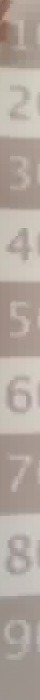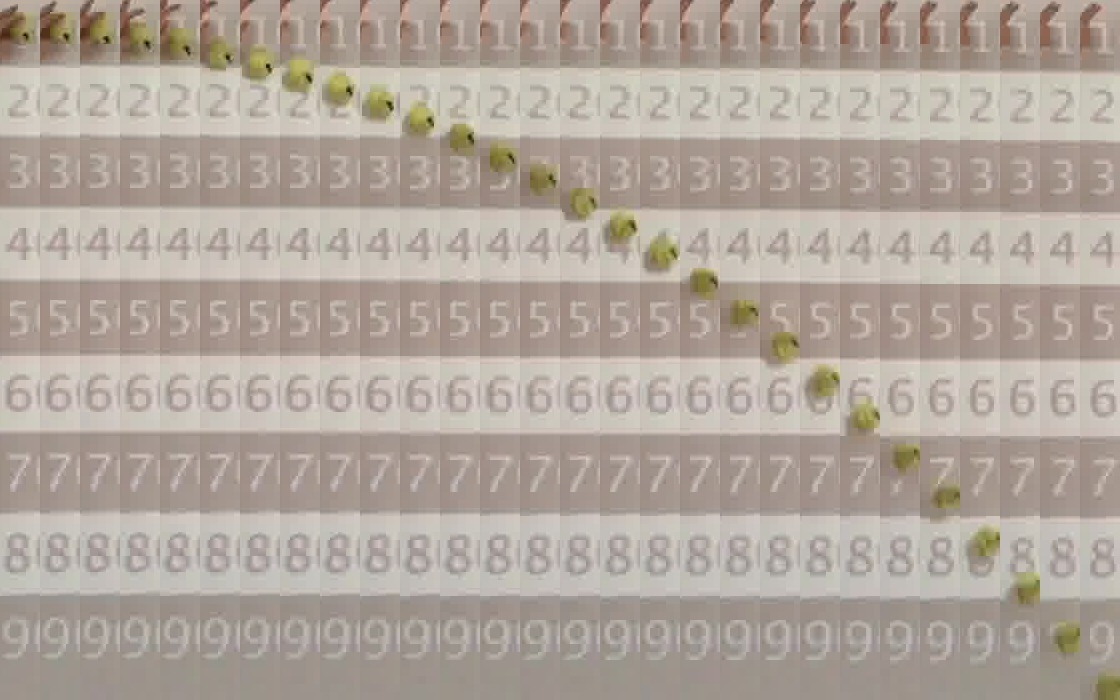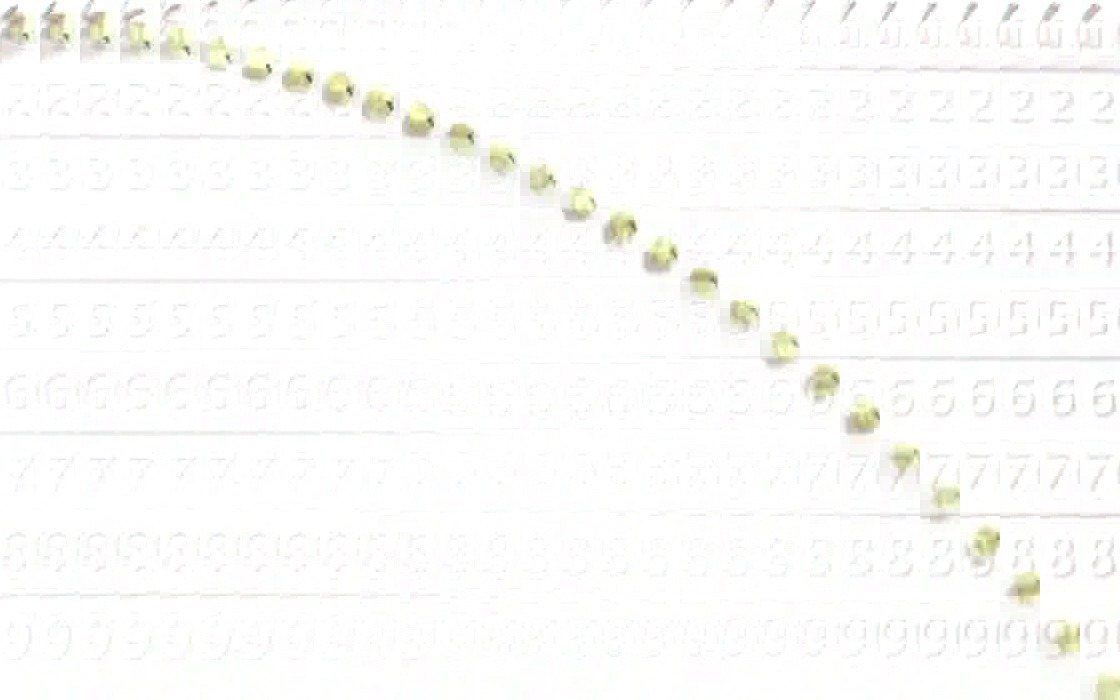Blog of Andrés Aravena

# Free fall experiment

10 December 2018

Note: Here we used classical command-line tools that are available on Linux, Mac, and other Unix based platforms, but not necessarily in Microsoft Windows. It is possible to do most of the analysis directly in R, as we will show in another post.

For the “Computing in Molecular Biology” course I try to use realistic data. It is difficult to do biological experiments during classes, since they require a lot of time. That is why I prefer to do physics experiments. Last week I did one of those experiments, to generate data that the students will analyze later.

With a bit of HTML and JavaScript, I made a web page with a stopwatch and a distance scale. The chronometer is controlled with the keyboard or with the arrows of the laser pointer. The students used their cell phones to record a video of a falling ball. I also made my video, and I uploaded it to YouTube. You can also see it here:

My video starts at normal speed for a couple of seconds, and then records in slow motion. The YouTube video can be played at a quarter of the normal speed, allowing you to see and note when the ball crosses the marks of the ladder. The clock did not work as I expected, probably because I forgot to close the other processes on the computer. An alternative for the next time is to use an external display controlled by an Arduino.

# Extracting the interesting part

But not all is lost. In theory, video is recorded at a constant speed, so you can use the same video as a clock. Following a recipe that found on the internet, I used FFMPEG to create an image of each frame . The interesting information that FFMPEG delivers is:

Metadata:
major_brand     : qt
creation_time   : 2018-12-03T11:06:29.000000Z
com.apple.quicktime.make: Apple
com.apple.quicktime.model: iPhone SE
Duration: 00:00:12.14, start: 0.000000, bitrate: 8981 kb/s
Stream #0:1(und): Video: h264 (High) (avc1 / 0x31637661),
yuv420p(tv, bt709), 1280x720, 8792 kb/s,
30 fps, 30 tbr, 600 tbn, 1200 tbc (default)
encoder         : H.264

At 30 frames/second during 12.14 seconds, 365 images are obtained. Reviewing frame by frame I decided that the most interesting thing happens between frames 110 and 245.

To study the fall, only a column of 40 or 50 pixels wide is needed. To manipulate images you can use the convert program of Imagemagik, which can do many things from the command line. For example the command

convert frame0110.jpg -crop 40x700+310+20 col0110.jpg

generates a 40x700 pixel image, right where the ball moves. Using a for cycle you can do the same for each frame.

for i in $(seq -w 365) do convert frame0$i.jpg -crop 40x1100+310+20 col$i.jpg done Then everything is put together using the command convert col0???.jpg +append composite.jpg and we get this image To limit the image to a reasonable size, you can use convert$(seq -w 110 5 245 |sed 's/$$.*$$/col\1.jpg/') +append composite.jpg

, which already seems to tell us something:Background image to isolate the ball# Isolating the image of the ball

Since we have the tools, we can use the computer to measure the distance of the ball to the top edge in each frame. The ideal is to isolate the ball and separate it from the background. For that we need a background reference. Most of the time the ball is not at any fixed point, so we can build a “background image” taking the median of each frame:

convert col0???.jpg -evaluate-sequence median bgr.jpg

The resulting image is blurred, because I did not use a tripod. Next time you have to use tripod The command convert -evaluate-sequence median reported memory handling errors, but they were not reproducible. Repeating the command several times, I eventually got the image shown here.

.

Now we can isolate the ball in each frame, using a for cycle like this:

for i in $(seq -w 110 245) do convert col$i.jpg bgr.jpg -compose minus -composite diff$i.jpg done convert diff*.jpg +append -negate composite-diff.jpg and we get this:Now we can extract the yellow channel from each diff * .jpg file and convert it to text. For this we use convert once more, and we choose the PGM format for the standard output. There are 4 header lines and then one line of text for each line in the image. Using gawk we calculate the median of each line. for i in$(seq -w 110 245)
do
convert diff$i.jpg -channel Y -separate -compress none \ -depth 8 PGM:- | gawk 'NR>4 {split($0,b); asort(b,a); \
print a[int(NF/2)]}' > median$i.txt done paste median*.txt > median.tsv Now the file median.tsv contains the intensity of yellow in each line of each frame. We process this file with R. # Automatic location of the ball In R we can read our data and make a simple graph signal <- read.delim("median.tsv", header=FALSE) frames <- 1:ncol(signal) pixels <- 1:nrow(signal) opar=par(mfrow=c(1,2), mar=c(5,4,0.5,0.5)) plot(pixels, pixels, type="n", ylab="pixels", xlab="frame", xlim=range(frames)) for(i in frames) { lines(0.1*signal[[i]]+i, rev(pixels)) } image(x=frames, y=pixels, z=t(signal)[,rev(pixels)], useRaster = TRUE, col = terrain.colors(200))The signal matrix has one column for each frame and one row for each pixel on the vertical axis. In principle we can find the location of each peak using the function which.max() in each column, but the result is not very good.fall <- data.frame(frames, y = -apply(signal[30:700,], 2, which.max)) plot(y ~ frames, data=fall) We can reduce noise by taking a mobile median filter. Now the ball location looks much better.clean_signal <- sapply(frames, function(i) runmed(signal[[i]], k=11)) fall$px <- -apply(clean_signal[30:700,], 2, which.max)
plot(px ~ frames, data=fall)
model_px <- lm(px ~ frames + I(frames^2), data=fall)
lines(predict(model_px), col="red", lwd=2)

and we get a good fit to a second-degree polynomial model

summary(model_px)
Call:
lm(formula = px ~ frames + I(frames^2), data = fall)

Residuals:
Min      1Q  Median      3Q     Max
-11.123  -3.348   0.558   3.408   8.296

Coefficients:
Estimate Std. Error t value Pr(>|t|)
(Intercept) -5.791967   1.207905   -4.80  4.3e-06 ***
frames       0.271828   0.040705    6.68  6.0e-10 ***
I(frames^2) -0.036823   0.000288 -127.94  < 2e-16 ***
---
Signif. codes:  0 '***' 0.001 '**' 0.01 '*' 0.05 '.' 0.1 ' ' 1

Residual standard error: 4.63 on 133 degrees of freedom
Multiple R-squared:  0.999, Adjusted R-squared:  0.999
F-statistic: 1.2e+05 on 2 and 133 DF,  p-value: <2e-16

This proof of concept is limited by the units of measurement. We have pixels per frame, and we want meters per second. We need more information.

# How long is each frame?

In the original video, and in each image of a complete frame, you can see the chronometer. In theory you can use OCR to get that number. In practice it is easier to do it “by hand”, with the help of the computer.

Using JavaScript I made a web page that shows each frame one by one, and presents an input box where you can type the number that is read in the photo. If the number does not change, the entry can be left blank. The JavaScript program collects the data and allows you to download them at the end. I got the file read-20181208T0857.txt, which we can read in R.

f <- read.delim("read-20181208T0857.txt")

The file has three columns: frame, file, and millisec. Several lines have the field millisec empty, and we can eliminate them. We also eliminate duplicate lines.

f <- subset(f, !is.na(millisec))
f <- subset(f, !duplicated(millisec))
plot(millisec ~ frame, f)It is clear that the first frames are at a different speed than the last ones. To find the exact point we can evaluate the “derivative”.

ms_frame <- diff(f$millisec)/diff(f$frame)
plot(ms_frame)
abline(h=10)We see that all the first frames take more than 10 milliseconds. We use that value as a cutoff point.

thr  <- min(f$frame[ms_frame <10]) plot(ms_frame, type="n") text(ms_frame, labels=f$frame, cex=0.5)
abline(v=which(f$frame==thr))This means that frame 31 is the first one in slow motion. We use that limit to build a linear model. model_time <- lm(millisec~frame, f, subset=frame>=thr) summary(model_time) Call: lm(formula = millisec ~ frame, data = f, subset = frame >= thr) Residuals: Min 1Q Median 3Q Max -16.68 -4.36 -0.12 7.37 13.12 Coefficients: Estimate Std. Error t value Pr(>|t|) (Intercept) 1.79e+03 2.50e+00 717 <2e-16 *** frame 4.17e+00 1.16e-02 359 <2e-16 *** --- Signif. codes: 0 '***' 0.001 '**' 0.01 '*' 0.05 '.' 0.1 ' ' 1 Residual standard error: 8.28 on 52 degrees of freedom Multiple R-squared: 1, Adjusted R-squared: 1 F-statistic: 1.29e+05 on 1 and 52 DF, p-value: <2e-16 That is, each frame has a duration of 4.168 milliseconds, on average. More precisely, the duration is inside the following interval 1000/confint(model_time, level=0.99)  0.5 % 99.5 % (Intercept) 0.559 0.555 frame 241.740 238.170 Now we can add a column with time in milliseconds of each frame. To simplify the following analysis we are going to set the first frame as time 0. fall$time <- (fall$frames-1)*coef(model_time)["frame"] # Measuring the vertical distance Our distance data is expressed in pixels. We need to convert it to meters. First let’s measure the distance between each black and white band. We graph the original signal of the last frame, without any filter.last_frame <- signal[ , ncol(signal)] plot(last_frame, type="l") abline(h=25) There are several peaks equally spaced (or almost). These correspond to the transitions between the colors of the bands. If I had used a tripod they would not be seen. In this case the small movement of the camera is an advantage. Taking a cut at level 25, we can group similar values into a few groups.px <- which(last_frame>25 & last_frame<50) hist(px, nclass=20, col="grey") There are 5 natural groups. We assign labels to each one of thembar <- as.numeric(cut(px, 5)) plot(bar~px) This means that the correlation between pixels and bars is reasonably linear. The only extra data we need is the size of each bar in centimeters. I did not measure it carefully, I’ll do better next time. A black bar in the center measured between 18 and 19 centimeters. The peaks of this model are every two bars, that is, every 36cm. We incorporate that data in our distance model.mtr <- bar*0.36 model_dist <- lm(mtr ~ px) plot(mtr ~ px) abline(model_dist) summary(model_dist) Call: lm(formula = mtr ~ px) Residuals: Min 1Q Median 3Q Max -0.02020 -0.01577 0.00323 0.01247 0.02428 Coefficients: Estimate Std. Error t value Pr(>|t|) (Intercept) 2.08e-01 8.10e-03 25.7 3.5e-13 *** px 2.37e-03 1.93e-05 122.7 < 2e-16 *** --- Signif. codes: 0 '***' 0.001 '**' 0.01 '*' 0.05 '.' 0.1 ' ' 1 Residual standard error: 0.0168 on 14 degrees of freedom Multiple R-squared: 0.999, Adjusted R-squared: 0.999 F-statistic: 1.51e+04 on 1 and 14 DF, p-value: <2e-16 # Final Model Now we are in a position to put everything together. We add a column with vertical position in meters and another with time in seconds. To calculate the second degree polynomial model, we add another column with time squared. fall$y_m <- predict(model_dist, newdata=data.frame(px=fall$px)) fall$t_sec <- fall$time/1000 fall$t_sec2 <- fall\$t_sec^2

Now we make the final model.

model_final <- lm(y_m ~ t_sec + t_sec2, data=fall)
plot(y_m ~ t_sec, data=fall)
lines(predict(model_final, newdata=fall)~ t_sec, data=fall, col="red", lwd=2)summary(model_final)
Call:
lm(formula = y_m ~ t_sec + t_sec2, data = fall)

Residuals:
Min       1Q   Median       3Q      Max
-0.02641 -0.00795  0.00133  0.00809  0.01970

Coefficients:
Estimate Std. Error t value Pr(>|t|)
(Intercept)  0.19495    0.00278   70.01  < 2e-16 ***
t_sec        0.11290    0.02287    4.94  2.3e-06 ***
t_sec2      -5.03312    0.03934 -127.94  < 2e-16 ***
---
Signif. codes:  0 '***' 0.001 '**' 0.01 '*' 0.05 '.' 0.1 ' ' 1

Residual standard error: 0.011 on 133 degrees of freedom
Multiple R-squared:  0.999, Adjusted R-squared:  0.999
F-statistic: 1.2e+05 on 2 and 133 DF,  p-value: <2e-16

According to this, the acceleration of gravity is $$10m/s^2.$$ A little bit much. I suspect that:

• You have to have a better watch
• You have to measure distances more carefully

The real challenge is to remake everything, keeping track of the margins of error in each step.